# Statistics for Psychology, 6th Edition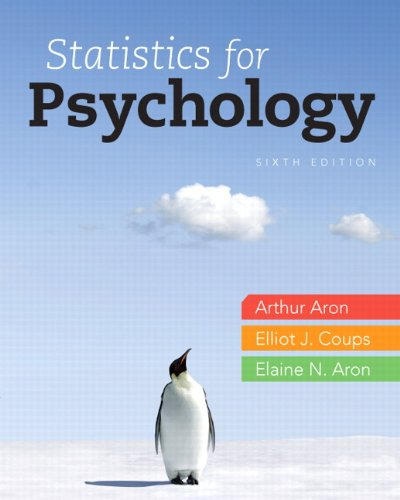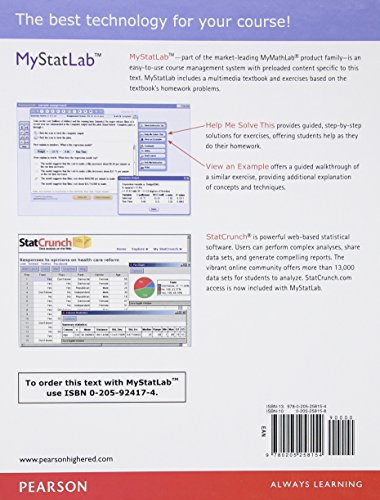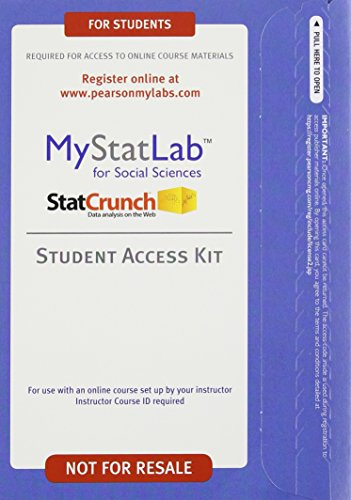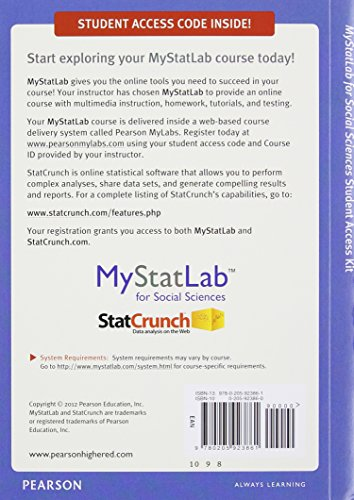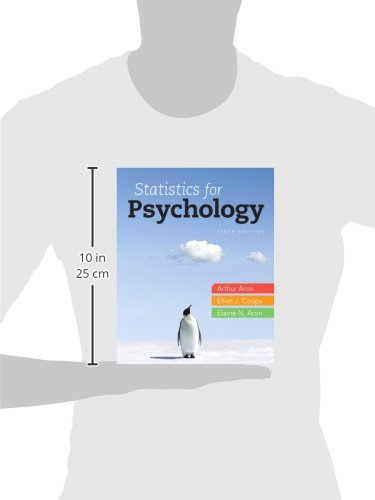Emphasizing meaning and concepts, not just symbols and numbers

Statistics for Psychology, 6th edition places definitional formulas center stage to emphasize the logic behind statistics and discourage rote memorization. Each procedure is explained in a direct, concise language and both verbally and numerically.

MyStatLab is an integral part of the Statistics course. MyStatLab gives students practice with hundreds of homework problems. Every problem includes tools to help students understand and solve each problem – and grades all of the problems for instructors. MyStatLab also includes tests, quizzes, eText, a Gradebook, a customizable study plan, and much more.

Learning Goals

Upon completing this book, readers should be able to:

• Know both definitional and numerical formulas and how to apply them
• Understand the logic behind each formula
• Expose students to the latest thinking in statistical theory and application
• Prepare students to read research articles
• Learn how to use SPSS

Note: This is the standalone book if you want the book/access card please order the ISBN below;

0205924174 / 9780205924172 Statistics for Psychology Plus NEW MyStatLab with eText — Access Card Package

Package consists of:

0205258158 / 9780205258154 Statistics for Psychology

0205923860 / 9780205923861 New MyStatLab for Social Sciences with Pearson eText — ValuePack Access Card

Used Book in Good Condition